# Neural Art Style Transfer with Keras — Theory and Implementation

Source: Deep Learning on Medium

Introduction

Over the past five years, neural networks have received attention through AI-generated art pieces, whether these be paintings, poetry, or music. During October of last year, an AI-generated art piece sold for over \$400,000 at an auction at Christie’s, sparking debate and discussion over the intrinsic value and nature of art generated by machines.

While most of these mentioned art pieces were original pieces generated through Generative Adversarial Networks (GAN’s, which we will discuss in a future tutorial), apps such as PRISMA have been receiving attention for being able to apply the styles of famous paintings to one’s own photos. The concept, known as neural style transfer (henceforth NST), was first introduced in a paper by Leon Gatys et al. in 2015, and more recently was implemented as a part of Tensorflow’s demo app showcase.

NST uses a trained Neural Network to take target image and a reference image, and produce an output which retains both the content of the target image along with the style of the reference image. We can best illustrate this in the example below — here, the target image is an MRI scan , and the reference image the (1832) painting of the Great Wave off Kanagawa.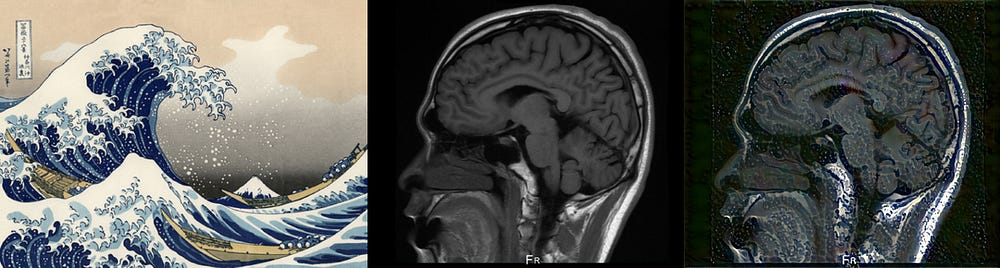The Great Wave off Kanagawa, a brain MRI, and the resulting composite image after 4 iterations, at a style/content ratio of 3200.

In this tutorial, we will use VGG19 network architecture, pre-trained on over a million images for image classification tasks, to perform style transfer using the Keras framework. Our code is adapted from Francois Chollet’s excellent Deep Learning with Python reference, where the subject was briefly covered in chapter 8. We assume that the reader is familiar with the elements of deep learning, particularly with loss functions and backpropagation training. Those looking for a quick refresher are encouraged to audit Andrew Ng’s original Machine Learning course, which goes into deeper details over the operation and structure of neural networks.

Theory

During NST, we define two losses in order to preserve both the content of the target image and the style of the reference image. The loss function is a weighted sum of the content loss and style loss which is minimized using gradient descent. Intuitively, we are iteratively updating our output image in such a way that it minimizes our total loss by bringing the output as close as possible to the content of the target image and the style of the reference image.

So how do we define content and style losses? Recall that during image classification, a neural network’s earlier layers captures the lower-level features, with later layers focusing on identifying more complex patterns, with the eventual aim of producing a classification output.

We hence define content loss simply as the L2 distance between the intermediate content representations , taken from higher (later) layers of a pre-trained neural network, for a input image and the target image. As a high level layer produces filters that possess complex raw information for the input image, this is a suitable approximation for judging similarity in terms of content. The equation is shown below: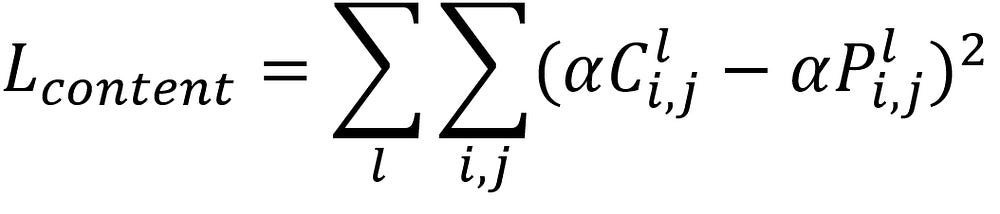Equation for the Content Loss, defined as the squared-error loss between the two feature representations of the target (C) and output (P) images in a particular layer l.

Similarly, we define the style loss as the L2 distance between the gram matrices of the intermediate style representations for the style image (taken from lower layers of a pre-trained neural network) and the output images. The lower level layers capture more simple image features which best encode the concept of style. Intuitively, Gram matrices we distribute and delocalize spatial information in an image and approximate the “style” of an image. Mathematically, they are matrices are simply multiplication of the image matrix and its transpose.Equation for the Gram Matrix. G is the inner product between the vectorised feature maps i and j in a particular layer l.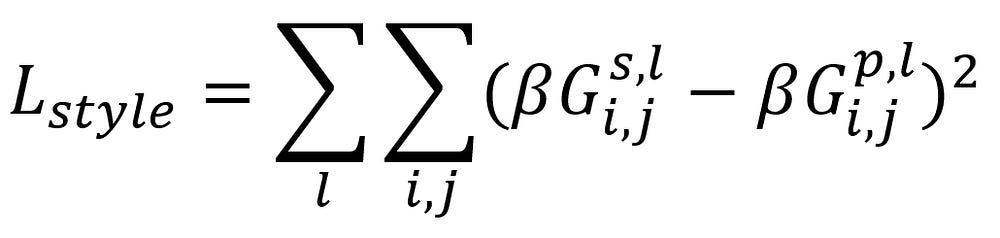Equation for the Style Loss, defined as the squared-loss error between the gram matrices of the style reference (s) and the output image (p), over a subset of particular layers.

Finally, we add a third loss value known as the total variation loss (TVL) . While not seen in the original paper, TVL was introduced in a paper by Mahendran and Vedaldi in 2015 with the aim to encourage image consistency and special continuity, minimizing pixilation and sharp feature formation. TVL works by penalizing larger gradients during the transfer process, distributing overall changes across larger regions rather than concentrating them at points or curves, ensuring a smoother image at the expense of image sharpness.

To conclude, by summing up and minimizing all three aforementioned losses, we generate an output image that best matches the content of our target image, while adopting the new style from the reference image.

Implementation

Now that the concepts have been cleared up, let’s take a look at the code itself. We are using the Keras library, so make sure that’s installed before you begin.

First off, let’s load our packages and define some variables, as well as our input and output sizes. Note that the larger images will take a longer time to generate.

`from keras.preprocessing.image import load_img, img_to_arrayfrom keras import backend as K`
`target_image_path = 'input/styletransfer/brainMRI.jpg'reference_image_path = 'input/styletransfer/kanagawa.jpg'`
`width, height = load_img(target_image_path).sizeimg_height = 800img_width = int(width * img_height / height)`

Next, let’s implement some auxiliary functions to preprocess our images for input to the VGG19 network. The deprocess function converts the processed image into its original form for later visualization.

`import numpy as np`
`def preprocess_image(image_path):    img = load_img(image_path, target_size=(img_height, img_width))    img = img_to_array(img)    img = np.expand_dims(img, axis=0)    img = vgg19.preprocess_input(img)    return img`
`def deprocess_image(x):    x[:, :, 0] += 103.939    x[:, :, 1] += 116.779    x[:, :, 2] += 123.68    x = x[:, :, ::-1]    x = np.clip(x, 0, 255).astype('uint8')    return x`

Now, let’s load up our pre-trained VGG19 neural network model and feed it our input tensors — namely, the target image, the style reference, and an output image, which we initialize as an appropriately sized placeholder filled with white noise. As other pre-trained networks rely on different datasets, we encourage you to try out other architectures as well. Note that we concatenate all three images into a single input tensor by treating them as a batch of images and not individual inputs.

`from keras.applications import vgg19`
`target_image = K.constant(preprocess_image(target_image_path))reference_image = K.constant(preprocess_image(reference_image_path))combination_image = K.placeholder((1, img_height, img_width, 3))`
`input_tensor = K.concatenate([target_image,                              reference_image,                              combination_image], axis=0)model = vgg19.VGG19(input_tensor=input_tensor,                    weights='imagenet',                    include_top=False)`

With all of the preparatory work finished, let’s define the content, style, and total variation losses. Additionally, we define the mathematical implementation of the gram matrix in our code.

`def content_loss(base, combination):    return K.sum(K.square(combination - base))`
`def gram_matrix(x):    features = K.batch_flatten(K.permute_dimensions(x, (2, 0, 1)))    gram = K.dot(features, K.transpose(features))    return gram`
`def style_loss(style, combination):    S = gram_matrix(style)    C = gram_matrix(combination)    channels = 3    size = img_height * img_width    return K.sum(K.square(S - C)) / (4. * (channels ** 2) * (size ** 2))`
`def total_variation_loss(x):    a = K.square(            x[:, :img_height - 1, :img_width - 1, :] -            x[:, 1:, :img_width - 1, :])    b = K.square(            x[:, :img_height - 1, :img_width - 1, :] -            x[:, :img_height - 1, 1:, :])    return K.sum(K.pow(a + b, 1.25))`

Recall that we calculate content and style losses from different levels of the VGG19 neural network. Let’s define these here, along with the weights of each respective loss toward the overall sum total loss. Feel free to play around with these values — a higher content/style ratio will yield an output image more representative of the original target image, while the opposite will yield an output image with stronger stylistic features.

`outputs_dict = dict([(layer.name, layer.output) for layer in model.layers])content_layer = 'block5_conv2'style_layers = ['block1_conv1',                'block2_conv1',                'block3_conv1',                'block4_conv1',                'block5_conv1']`
`total_variation_weight = 1e-5style_weight = 1.content_weight = 0.0003125`

Finally, we define the relationships between our variables and the VGG19 neural network through Keras, and begin summing up our losses. Note how the content loss is defined for one higher layer of the neural network only (‘block5_conv2’) , while the style loss is accumulated across several lower-level layers. This holds for our example, but you may want to play with the layer compositions to observe how the output changes.

`loss = K.variable(0.)layer_features = outputs_dict[content_layer]target_image_features = layer_features[0, :, :, :]combination_features = layer_features[2, :, :, :]`
`loss += content_weight * content_loss(target_image_features,                                      combination_features)for layer_name in style_layers:    layer_features = outputs_dict[layer_name]    reference_features = layer_features[1, :, :, :]    combination_features = layer_features[2, :, :, :]    sl = style_loss(reference_features, combination_features)    loss += (style_weight / len(style_layers)) * slloss += total_variation_weight * total_variation_loss(combination_image)`

Now with that complete, we wrap up by defining the overall methods that begin the loss calculation process. We fetch the gradients and loss for our output image using fetch_loss_and_grads. We then use gradient descent to minimize our defined loss and update the gradients, which will ensure maximum similarity between the contents of our target and output images, and the styles of our reference and output images.

`grads = K.gradients(loss, combination_image)fetch_loss_and_grads = K.function([combination_image], [loss, grads])`

Finally, we finish by defining and initializing the overall evaluator classes to kickstart the training process. We use Keras’s built-in BFGS optimizer class for gradient descent, over 20 iterations. We also save our images after each epoch for inspection.

`class Evaluator(object):    def __init__(self):        self.loss_value = None        self.grads_values = None    def loss(self, x):        assert self.loss_value is None        x = x.reshape((1, img_height, img_width, 3))        outs = fetch_loss_and_grads([x])        loss_value = outs        grad_values = outs.flatten().astype('float64')        self.loss_value = loss_value        self.grad_values = grad_values        return self.loss_value`
`def grads(self, x):        assert self.loss_value is not None        grad_values = np.copy(self.grad_values)        self.loss_value = None        self.grad_values = None        return grad_values`
`evaluator = Evaluator()`
`from scipy.optimize import fmin_l_bfgs_bfrom scipy.misc import imsaveimport time`
`result_prefix = 'my_result'iterations = 20x = preprocess_image(target_image_path)x = x.flatten()for i in range(iterations):    print('Start of iteration', i)    start_time = time.time()    x, min_val, info = fmin_l_bfgs_b(evaluator.loss,                                     x,                                     fprime=evaluator.grads,                                     maxfun=20)    print('Current loss value:', min_val)    img = x.copy().reshape((img_height, img_width, 3))      img = deprocess_image(img)    fname = result_prefix + '_at_iteration_%d.png' % i    imsave(fname, img)    print('Image saved as', fname)    end_time = time.time()    print('Iteration %d completed in %ds' % (i, end_time - start_time))`

Running the code, you should be able to visualize iterative evolutions of your image, in a style similar to below (iterations 1 through 20):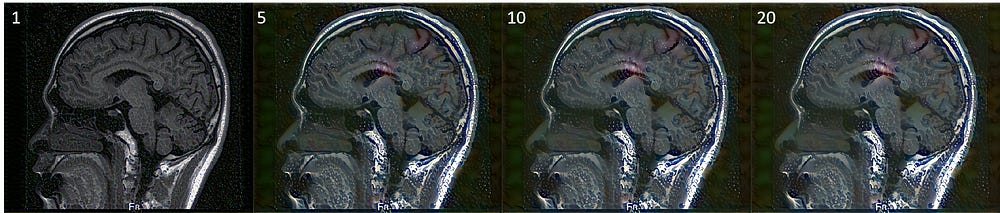Iterative evolution of the original target image as it undergoes style transfer.

Note how the features of the stylistic image become stronger with time, particularly along sharply defined, high-contrast elements of the image. These juxtapose against the more muddled colors that take place of the original black background, which may have been inferred from the background of the original painting.

I encourage you to run the code with your own images, and play with the weights to achieve interesting results. To finish, below you can see a collage of of the same MRI with multiple different styles applied to it.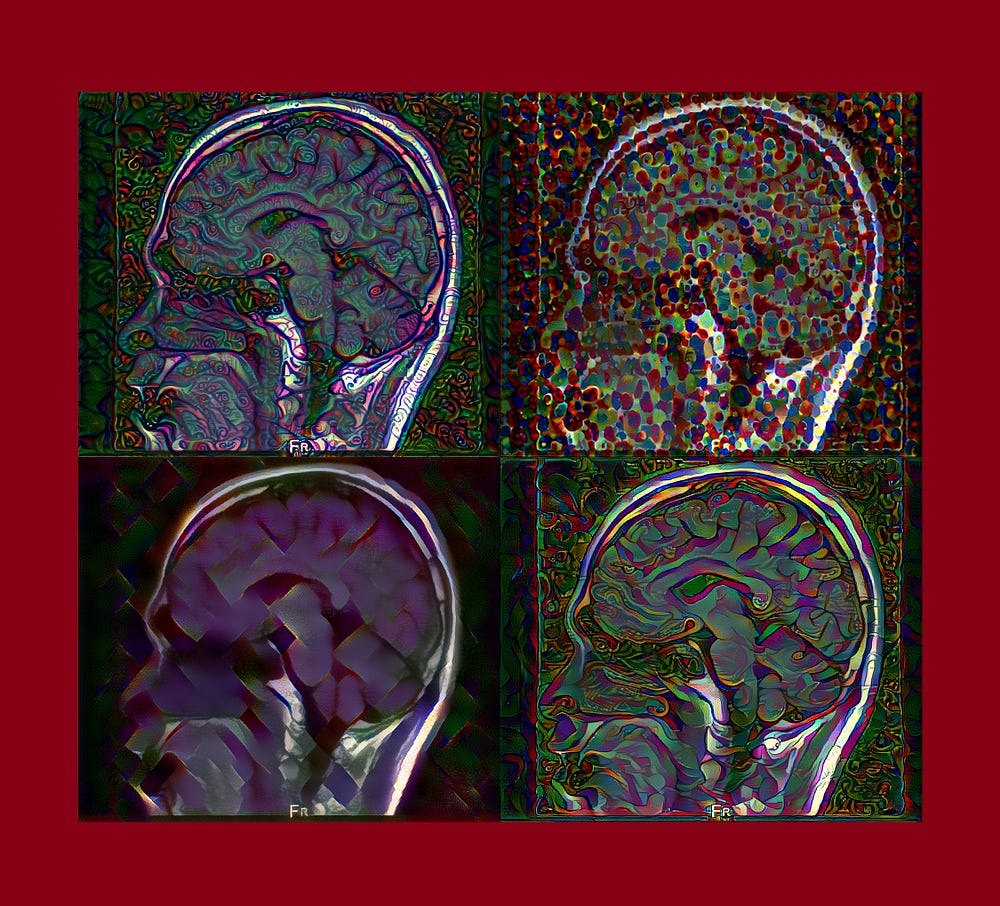Collage of 4 brain MRI instances that underwent style transfer using 4 separate style references.

We hope you’ve found this tutorial interesting and fun. Next time, we automate the generation of ancient script through Generative Adversarial Networks!

References

Gatys et al., A Neural Algorithm of Artistic Style

Chollet, Deep Learning with Python